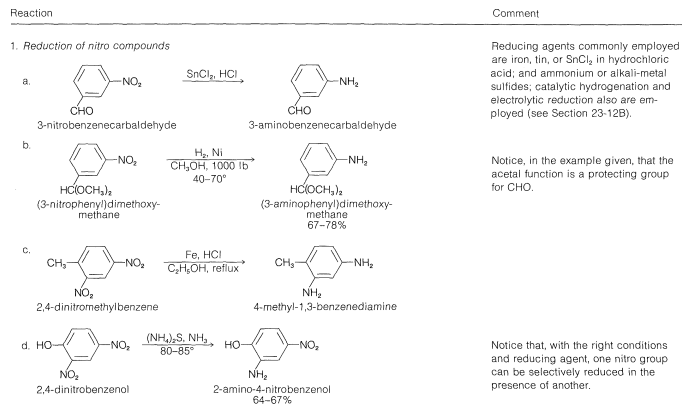# 23.12: Synthesis of Amines

$$\newcommand{\vecs}{\overset { \rightharpoonup} {\mathbf{#1}} }$$ $$\newcommand{\vecd}{\overset{-\!-\!\rightharpoonup}{\vphantom{a}\smash {#1}}}$$$$\newcommand{\id}{\mathrm{id}}$$ $$\newcommand{\Span}{\mathrm{span}}$$ $$\newcommand{\kernel}{\mathrm{null}\,}$$ $$\newcommand{\range}{\mathrm{range}\,}$$ $$\newcommand{\RealPart}{\mathrm{Re}}$$ $$\newcommand{\ImaginaryPart}{\mathrm{Im}}$$ $$\newcommand{\Argument}{\mathrm{Arg}}$$ $$\newcommand{\norm}{\| #1 \|}$$ $$\newcommand{\inner}{\langle #1, #2 \rangle}$$ $$\newcommand{\Span}{\mathrm{span}}$$ $$\newcommand{\id}{\mathrm{id}}$$ $$\newcommand{\Span}{\mathrm{span}}$$ $$\newcommand{\kernel}{\mathrm{null}\,}$$ $$\newcommand{\range}{\mathrm{range}\,}$$ $$\newcommand{\RealPart}{\mathrm{Re}}$$ $$\newcommand{\ImaginaryPart}{\mathrm{Im}}$$ $$\newcommand{\Argument}{\mathrm{Arg}}$$ $$\newcommand{\norm}{\| #1 \|}$$ $$\newcommand{\inner}{\langle #1, #2 \rangle}$$ $$\newcommand{\Span}{\mathrm{span}}$$

## Main Types of Synthesis

There are seemingly many different ways in which amines can be prepared. However, a careful look at these methods reveals that they fall into three main groups of reactions. The first group starts with a simple amine, or with ammonia, and builds up the carbon framework by alkylation or arylation reactions on nitrogen, as discussed in Section 23-9D:

$\ce{RX} + \ce{NH_3} \rightarrow \ce{RNH_2} + \ce{HX}$

The second group starts with compounds of the same carbon-nitrogen framework as in the desired amine but with nitrogen in a higher oxidation state. The amine then is obtained from these compounds by catalytic hydrogenation or metal-hydride reduction, as will be described in the next section:

$\ce{RNO_2} + 3 \ce{H_2} \overset{\ce{Pt}}{\longrightarrow} \ce{RNH_2} + 2 \ce{H_2O}$

The third group of reactions relies on the fact that amides usually can be converted to amines, either by reduction, hydrolysis, or rearrangement, so that any viable synthesis of amides usually is also a synthesis of amines:These and related reactions are discussed in further detail in the following sections. For your convenience, a tabular summary of methods for the synthesis of amines appears in Tables 23-6 and 23-7.

Table 23-6: Practical Examples of the Synthesis of AminesTable 23-7: Practical Examples of the Synthesis of Aromatic Amines## Formation of Amines by Reduction

Excellent procedures are available for the preparation of primary, secondary, and tertiary amines by the reduction of a variety of nitrogen compounds. Primary amines can be obtained by hydrogenation or by lithium aluminum hydride reduction of nitro compounds, azides, imines, nitriles, or unsubstituted amides [all possible with $$\ce{H_2}$$ over a metal catalyst ($$\ce{Pt}$$ or $$\ce{Ni}$$) or with $$\ce{LiAlH_4}$$]:Some care must be exercised in the reduction of nitro compounds because such reductions can be highly exothermic. For example, the reaction of $$1 \: \text{mol}$$ $$\left( 61 \: \text{g} \right)$$ of nitromethane with hydrogen to give methanamine liberates sufficient heat to increase the temperature of a $$25$$-$$\text{lb}$$ iron bomb $$100^\text{o}$$:

Secondary and tertiary amines, particularly those with different $$\ce{R}$$ groups, are prepared easily by lithium aluminum hydride reduction of substituted amides (Section 18-7C).

## Amines by Reductive Alkylation of Aldehydes and Ketones

A useful synthesis of primary and secondary amines that is related to the reductions just described utilizes the reaction of an aldehyde or a ketone with ammonia or a primary amine in the presence of hydrogen and a metal catalyst:

\begin{align} \ce{RCHO} + \ce{NH_3} + \ce{H_2} &\overset{\ce{Pt}}{\longrightarrow} \ce{RCH_2NH_2} + \ce{H_2O} \\ \ce{R_2C=O} + \ce{CH_3NH_2} + \ce{H_2} &\overset{\ce{Pd}}{\longrightarrow} \ce{R_2CHNHCH_3} + \ce{H_2O} \end{align}

It is reasonable to suppose that the carbonyl compound first forms the imine derivative by way of the aminoalcohol (see Section 16-4C), and this derivative is hydrogenated under the reaction conditions:Other reducing agents may be used, and the borohydride salt $$\ce{Na}^\oplus \: ^\ominus \ce{BH_3(CN)}$$ is convenient to use in place of $$\ce{H_2}$$ and a metal catalyst.

In a formal sense, the carbonyl compound is reduced in this reaction while the amine is alkylated, hence the term reductive alkylation or reductive amination.

## Amines from Amides by Hydrolysis or Reduction

There are a number of ways in which an amide can be transformed into an amine. Two of these ways have been mentioned already and involve hydrolysis or reduction:As a means of amine synthesis, both methods depend on the availability or the ease of synthesis of the corresponding amide.

## Amines from Amides by the Hofmann Degradation

An interesting and general reaction for the preparation of primary amines is the Hofmann degradation, in which an unsubstituted amide is converted to an amine by bromine (or chlorine) in sodium hydroxide solution:The mechanism of this unusual reaction first involves base-catalyzed bromination of the amide on nitrogen to give an $$\ce{N}$$-bromoamide intermediate:There follows a base-induced elimination of $$\ce{HBr}$$ from nitrogen to form a "nitrene" intermediate, which is analogous to the formation of a carbene (Section 14-7B):As you might expect from the structure of an acyl nitrene (only six electrons in the valence shell of nitrogen), it is highly unstable but can become stabilized by having the substituent group move as $$\ce{R}^\ominus$$ from carbon to nitrogen:$$^5$$

The rearrangement is stereospecific and the configuration at the migrating carbon is retained (see Section 21-10F). The rearrangement product is called an isocyanate and is a nitrogen analog of a ketene $$\left( \ce{R_2C=C=O} \right)$$; like ketenes, isocyanates readily add water. The products are carbamic acids, which are not very stable, especially in basic solution, and readily lose carbon dioxide to give the amine:A practical example of this reaction is given in Table 23-6 together with examples of related reactions known as the Curtius and Schmidt rearrangements. The latter two probably also involve rearrangement of an acyl nitrene, this time formed by decomposition of an acyl azide:$$^5$$There are several analogies for this kind of rearrangement that involve electron-deficient carbon (Sections 8-9B and 15-5E) and oxygen (Section 16-9E).